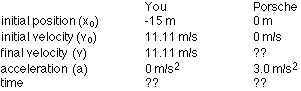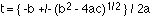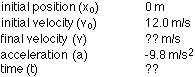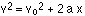## Constant Acceleration

9-10-99

Sections 2.6 - 2.7

### Applying the equations

Doing a sample problem is probably the best way to see how you would use the kinematics equations. Let's say you're driving in your car, approaching a red light on Commonwealth Avenue. A black Porsche is stopped at the light in the right lane, but there's no-one in the left lane, so you pull into the left lane. You're traveling at 40 km/hr, and when you're 15 meters from the stop line the light turns green. You sail through the green light at a constant speed of 40 km/hr and pass the Porsche, which accelerated from rest at a constant rate of 3 m/s2 starting at the moment the light turned green.

(a) How far from the stop line do you pass the Porsche?

(b) When does the Porsche pass you?

(c) If a Boston police officer happens to get you and the Porsche on the radar gun at the instant the Porsche passes you, will either of you be pulled over for speeding? Assume the speed limit is 50 km/hr.

Step 1 - Write down everything you know. Define an origin - the stop line is a good choice in this problem. Then choose a positive direction. In this case, let's take the positive direction to be the direction you're traveling. Decide on a system of units...meters and seconds is a good choice here, so convert your speed to m/s from km/hr. Drawing a diagram is also a good idea.

Origin = stop line
Positive direction = the direction you're travelingStep 2 - Figure out what you need to solve for. At the instant you pass the Porsche, the x values (yours and the Porsche's) have to be equal. You're both the same distance from the stop line, in other words. Write out the expression for your x-value and the Porsche's. We'll use the equation:For you : x = -15 + 11.11 t + 0

For the Porsche : x = 0 + 0 + 1/2 (3) t2 = 1.5 t2

At some time t, when you pass the Porsche, these x values will be the same. So, we can set the equations equal to one another and solve for time, and then plug the time back in to either x equation to get the distance from the stop line. Doing this gives:

-15 + 11.11 t = 1.5 t2

Bringing everything to one side gives:

1.5 t2 - 11.11 t + 15 = 0

This is a quadratic equation, which we can solve using the quadratic formula:where a = 1.5, b = -11.11, and c = 15

This gives two values for t, t = 1.776 s and t = 5.631 s.

What do these two values mean? In many cases only one answer will be relevant, and you'll have to figure out which. In this case both are relevant. The smaller value is when you pass the Porsche, while the larger one is when the Porsche passes you back.

To get the answer to question (a), plug t = 1.776 into either of your x expressions. They should both give you the same value for x, so you can use one as a check.

For you, at t = 1.776, x = 4.73 m.
For the Porsche, at t = 1.776 s, x = 4.73 m.

We've actually already calculated the answer to (b), when the Porsche passes you, which is at t = 5.6 s.

To get the answer to part (c), we already know that you're traveling at a constant speed of 40 km/hr, which is under the speed limit. To figure out how fast the Porsche is going at t = 5.631 seconds, use:

v = vo + a t = 0 + (3) (5.631) = 16.893 m/s.

Converting this to km/hr gives a speed of 60.8 km/hr, so the driver of the Porsche is in danger of getting a speeding ticket.

### Free fall

Objects falling straight down under the influence of gravity are excellent examples of objects traveling at constant acceleration in one dimension. This also applies to anything you throw straight up in the air which, because of the constant acceleration downwards, will rise until the velocity drops to zero and then will fall back down again.

The acceleration experienced by a dropped or thrown object while it is in flight comes from the gravitational force exerted on the object by the Earth. If we're dealing with objects at the Earth's surface, which we usually are, we call this acceleration g, which has a value of 9.8 m/s2. This value is determined by three things: the mass of the Earth, the radius of the Earth, and a number called the universal gravitational constant. We'll be dealing with all that later in the semester, though, so don't worry about it yet. For now, all you need to remember is that g is 9.8 m/s2 at the surface of the Earth, directed down.

A typical one-dimensional free fall question (free fall meaning that the only acceleration we have to worry about is g) might go like this.

You throw a ball straight up. It leaves your hand at 12.0 m/s.

(a) How high does it go?

(b) If, when the ball is on the way down, you catch it at the same height at which you let it go, how long was it in flight?

(c) How fast is it traveling when you catch it?

Origin = height at which it leaves your hand
Positive direction = up(a) At the very top of its flight, the ball has an instantaneous velocity of zero. We can plug v = 0 into the equation:This gives:

0 = 144 + 2 (-9.8) x

Solving for x gives x = 7.35 m, so the ball goes 7.35 m high.

(b) To analyze the rest of the problem, it's helpful to remember that the down half of the trip is a mirror image of the up half. In other words, if, while going up, the ball passes through a particular height at a particular velocity (2 m/s up, for example), on its way down it will pass through that height at the same speed, with its velocity directed down rather than up. This means that the up half of the trip takes the same time as the down half of the trip, so we could just figure out how long it takes to reach its maximum height, and then double that to get the total time.

Another way to do it is simply to plug x = 0 into the equation:This gives 0 = 0 + 12 t - 4.9 t2

A factor of t can be canceled out of both terms, leaving:

0 = 12 - 4.9 t, which gives a time of t = 12 / 4.9 = 2.45 s.

(c) The answer for part (c) has to be 12 m/s down, because of the mirror-image relationship between the up half of the flight and the down half. We could also figure it out using the equation:

v = vo + a t

which gives:

v = 12 - 9.8 (2.45) = -12 m/s.